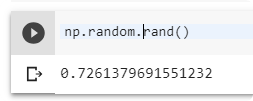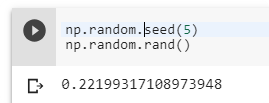# What does np.random.seed(2) do?

As the title of question reads, what exactly does the code; `np.random.seed(2)` do with respect to labeling(?) the data set? I’m also confused about the specific parameter passed to the method `seed()`, what would happen if it were some other number, say `0`, as described through examples in this Stack Overflow answer.

The concept of using seeds to make “predictable” random numbers is clear to me but the relevance of using it in that aspect seems pretty new to me.

EDIT: Found some possible solutions to the question;

4 Likes

It makes the the random block of the validation set data to be always the same. The block the function uses depends on the number you place inside seed(). If you put a different number inside the seed() parameter, then the function will use a different block.

2 Likes

@Mauro Alright! That’s something to start with. Could you direct me to the section of the documentation or the source code so that I could a look at it and know more on how it works out?

It’s a numpy method.

https://docs.scipy.org/doc/numpy/reference/generated/numpy.random.seed.html

1 Like

For a more thorough discussion of the issues in regard to fastai and PyTorch:

1 Like

Hi everyone, I am also having trouble understanding this line.

Mauro, when you say “random block of the validation set data” what exactly is the “random block”?

1 Like

The `ImageDataBunch` creates a validation set randomly each time the code block is run. To maintain a certain degree of reproducibility the `np.random.seed()` method is built-in within the `fastai` library.

What Mauro meant by, “random block of the validation set data” was that each time you might want to reproduce your code, `ImageDataBunch` would automatically choose a random chunk of data from the original dataset. This could be bad or good(you never know) according to your use case. Jeremy talks briefly in lesson 2 about it.

So in order to have some control and predictability in WHICH chunk of data should `ImageDataBunch` create the validation set from, the `np.random.seed()` is used.

9 Likes

Hi thanks @jarmos - I think I understand. It sounds like there is a specific set (or block) of images that the validation set gets randomly chosen from? So whenever I call that factory method to create a new image data bunch, the seed makes sure that we always choose from this same set of images? Instead of changing each time?

4 Likes

Yeah exactly!! You got it right this time around but I still don’t understand the significance of the number that’s passed as an argument to the `seed()` function. I mean why `2` specifically and not some other arbitrary number like say `9000`.

I asked around a couple of places but couldn’t get a proper explanation for it.

1 Like

It can be any number. Just that, when the same number is used again, the randomization is repeatable.
(so that the same set of images are picked by ImageDataBunch or in any other use of the randomizer, the numbers generated are repeated)

3 Likes

A random set or block of images. In this case the number of images inside this set or block depends on the length you assign to your validation set. If you have a total of 100 images, and you assign your validation set to be 20%, then your random block will have 20 random images from the 100 total.

2 Likes

Thanks everyone! I think I’m clear on most of that now. Although, still not clear though on the seed number. I understand now that not changing it keeps the block consistent between data bunch creation. But what does the actual number represent? Is it just some sort of id? Or is it numerically significant somehow?

I did some digging around. Here’s what I found from the NumPy documentation:

Seed the generator.

This method is called when `RandomState` is initialized. It can be called again to re-seed the generator. For details, see `RandomState` .

Parameters: seed : int or 1-d array_like, optional

So I checked the documentation for `RandomState` as well and here’s what I found:

Compatibility Guarantee A fixed seed and a fixed series of calls to ‘RandomState’ methods using the same parameters will always produce the same results up to roundoff error except when the values were incorrect.

I know it sounds confusing(at least to me) but from what I understand, is that, “a fixed seed” is necessary for `RandomState` to produce the same results every time. In other words once you execute the code block with `np.random.seed(2)`, DON’T change the seed argument to any other number if you want to reproducibility.

I also found a StackOverflow post - What does `numpy.random.seed(0)` do? which you can refer to understand the underlying mathematics involved, if you wanted to but I would strongly advice against it since it’s not relevant to the course.

(pseudo-)random numbers work by starting with a number (the seed), multiplying it by a large number, then taking modulo of that product. The resulting number is then used as the seed to generate the next “random” number. When you set the seed (every time), it does the same thing every time, giving you the same numbers.

1 Like

Note there are other randomisers in play for other libraries in use that you may want to set to constrain results further. Eg See Accumulating Gradients

Thanks jarmos. I’d still like to find out what the significance of the actual value is. I understand how changing it affects the reproducibility. But what is the difference between a 2 and say a 4 for the seed, or even some other arbitrary number like 10938? That’s kind of the place I’m stuck with on my understanding of that.

Seed is the starting point of the randomizing variables. Some initial number that computes next values by `np.random.rand()` function. Normally when you call function `np.random.rand()` the pseud-ogenerator generate you random number every time.But when you set up a seed the output of the function will be always the sameBased on this function the data set is divided between train and validation. You can set up this value to whatever you want, 2 is only as an example, but it must be the same value every time you run and load your DataSet to be sure that you will get the same output.

And also something interesting. You can even set a seed for torch.
`torch.manual_seed(2)`

After this, you will get the same results in your neural network,loss,accuracy and the same parameter values.

2 Likes

In short, nothing.

From https://stackoverflow.com/a/22639752, emphasis mine:

Pseudo-random number generators work by performing some operation on a value. Generally this value is the previous number generated by the generator. However, the first time you use the generator, there is no previous value.

Seeding a pseudo-random number generator gives it its first “previous” value.

2 Likes

Coincidentally even I was confused, for a while, with the choice of arguments for the `seed()` function. I gave up quickly since I realised that it’s taking me off-track and that’s something I wouldn’t spent much time on. But regardless here’s some resources I have had bookmarked since then.

Understanding the meaning of seed in generating random values?
This StackExchange answer gives a fairly straightforward answer assuming the reader has atleast high school level mathematical understanding.

Random Seed - Wikipedia

Pseudo-random number generators - Khan Academy

If you read up on these resources I believe it should be enough to get you on track to understand the underlying concepts of `np.random.seed()`. But REMEMBER these concepts aren’t relevant to the course in anyway and the more you dive in, the more you would get confused since then you would have brush up on mathematics and computer science concepts.

I see: so you are drawing the validation set from the original data set, and that validation set is determined at random? I get it in concept, but where does the generation of the validation data set (I am guessing it is ImageDataBunch) reference the seed? It is not a parameter passed to it.

The argument passed to `seed()` is quite complicated to explain and I don’t know the mathematics behind it either. All that I know is `np.random.seed(2)` yields a very different set of validation set if you pass `np.random.seed(198)` at some point of time.
The point is that the argument passed to `seed()` is arbitrary but it is important to bear in mind not to change it ever for future reproducibility.

1 Like